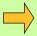"I proposed to him (Buxton) the following random question: In a body whose 3 sides are 23,145,789 yards, 5,642,732 yards, and 54,965 yards, how many cubical eighths of an inch? After once naming the several figures distinctly, one after another, in order to assure himself of the several dimensions and fix them in his mind, without more ado he fell to work amidst more than 100 of his fellow laborers, and after leaving him about 5 hours, on some necessary concerns (in which time I calculated it with my pen) at my return, he told me he was ready: upon which, taking out my pocket book and pencil, to note down his answer, he asked which end I would begin at, for he would direct me either way.... I chose the regular method........ and in a line of 28 figures, he made no hesitation nor the least mistake." Buxton also once found the amount obtained by doubling a farthing 140 times: his answer consisted of a 39-figure number for the pounds plus 2s 6d. Asked to multiply this 39-figure by itself he gave the answer after 2 months having calculated it from time to time over that period.

Jedediah Buxton was born in Derbyshire, England in 1702 and died there in 1772. Though his father was a schoolmaster he seems to have remained illiterate all his life and his achievements in mental calculation were the results of his own inventions. His memory, as is obvious from the examples above, was extraordinary, as also was his ability to measure area: "He will stride over a piece of land or a field, and tell you the contents of it, almost as exact as if you measured it by the chain..... He measured in this manner the whole lordship of Elmton, of some thousand acres."

Chapter 3

By One More Than The One Before

Here we see a special type of multiplication which is extremely fast and which occurs quite frequently in our calculations, especially when used in conjunction with the Proportionately formula from the previous chapter.

Squaring Numbers that End in 5

Squaring is multiplication in which a number is multiplied by itself: so 75 × 75 is called "75 squared" and is written 75².

The formula By One More Than the One Before provides a beautifully simple way of squaring numbers that end in 5.

In the case of 75², we simply multiply the 7 (the number before the 5) by the next number up, 8. This gives us 56 as the first part of the answer, and the last part is simply 25 (5²).Example 1

So 75² = 56/25 where 56=7×8, 25=5².Example 2

Similarly 65² = 4225:   42=6×7, 25=5².Example 3

And 25² = 625 where 6=2×3.Example 4

Also since 4½= 4.5, the same method applies to squaring numbers ending in ½.
So 4½² = 20¼, where 20 = 4×5 and ¼=½².

The method can be applied to numbers of any size:Example 5

305² = 93025 where 930 = 30×31.

Even for large numbers like, say, 635², it is still easier to multiply 63 by 64 and put 25 on the end than to multiply 635 by 635.

Question Comment !
 Tutorial Title Question Title No Questions 0 Questions Asked 0 Skipped Questions 0 Correctly Answered 0 Wrongly Answered 0 Total Question Attempts 0 Current Question Attempts 0

Skip Question

Reset Test

Halt Test

 No Questions 0 Questions Asked 0 Skipped Questions 0 Correctly Answered 0 Wrongly Answered 0 Total Question Attempts 0

Time Taken

CloseExercise A

Multiplying Numbers Whose First Figures are the Same and Whose Last Figures Add Up to 10, 100 etc.Example 6

Suppose we want to find 43 × 47 in which both numbers begin with 4 and the last figures (3 and 7) add up to 10.
The method is just the same as in the previous section: multiply 4 by the number One More: 4 × 5 = 20.
Then simply multiply the last figures together: 3 × 7 = 21.

So 43 × 47 = 2021,   (20 = 4×5, 21 = 3×7)Example 7

Similarly 62 × 68 = 4216,  (42 = 6×7, 16 = 2×8)Exercise B

The above technique can be extended using the Sutra Proportionaly:Example 8

88 × 46 may not look like it comes under this particular type of sum, but remembering the Proportionately formula from the previous chapter we notice that 88 = 2×44, and 44×46 does come under this type:

We know we can get 44 × 46 by this method and 88 × 46 is just twice that. So 44 × 46 = 2024 and therefore 88 × 46 = 2 × 2024 = 4048.Example 9

Similarly for 93 × 39 we notice that 93 = 3×31, and 31×39 does come under this type:

So 31 × 39 = 1209 (we put 09 as we need double figures here)

And so 93 × 39 = 3627 (multiply 1209 by 3)

The thing to notice is that the 39 needs a 31 for the method to work here: and then we spot that 93 is 3×31.Exercise C

This method can be extended in two further ways.Example 10

204 × 206: here both numbers start with 20, and 4 + 6 = 10, so the method applies here:

204 × 206 = 42024 (420 = 20×21, 24 = 4×6)Example 11

Finally, consider 397 × 303

Only the 3 at the beginning of each number is the same, but the rest of the numbers (97 and 03) add up to 100. So again the method applies, but this time we must expect to have four figures on the right-hand side:

397 × 303 = 120291,   (12 = 3×4, 0291 = 97×3).Exercise D